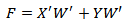# Karnaugh Mapping

## Karnaugh Mapping

Maurice Karnaugh, a telecommunications engineer, developed the Karnaugh map at Bell Labs in 1953 while designing digital logic based telephone switching circuits.

The Karnaugh mapping , like Boolean algebra, is a simplification tool applicable to digital logic.

The Karnaugh Map  simplifies the digital logic faster and more easily in most cases.

Boolean simplification is actually faster than the Karnaugh map for a task involving two or fewer Boolean variables. It is still quite usable at three variables, but a bit slower. At four input variables, Boolean algebra becomes tedious.

Karnaugh maps are both faster and easier. Karnaugh maps work well for up to six input variables and are usable for up to eight variables.

For more than six to eight variables, simplification should be done by CAD (computer automated design).

In this section we’ll examine some Karnaugh Maps for three and four variables and see how they are really being used to simplify Boolean functions.

• Draw the Karnaugh Map for the Boolean function.
• Use the information from a Karnaugh Map to determine the smallest sum-of-products function.

### 2-Variable Karnaugh Map

A Karnaugh map provides a pictorial method of grouping together expressions with common factors and therefore eliminating unwanted variables.

The Karnaugh map can also be described as a special arrangement of a truth table.

The diagram below illustrates the correspondence between the Karnaugh map and the truth table for the general case of a two variable problem.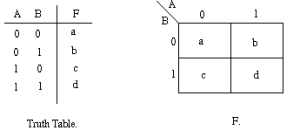The values inside the squares are copied from the output column of the truth table, therefore there is one square in the map for every row in the truth table.

Around the edge of the Karnaugh map are the values of the two input variable.

A is along the top and B is down the left hand side. The diagram below explains this: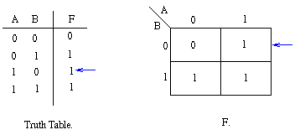The values around the edge of the map can be thought of as coordinates.

So as an example, the square on the top right hand corner of the map in the above diagram has coordinates A=1 and B=0. This square corresponds to the row in the truth table where A=1 and B=0 and F=1.

Note that the value in the F column represents a particular function to which the Karnaugh map corresponds.

Example 1:

Consider the following map. The function plotted is: Z = f(A,B) = A+ AB

Truth Table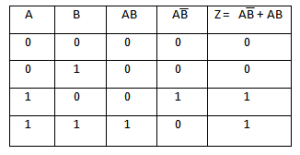Karnaugh-Map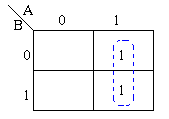• Note that values of the input variables form the rows and columns. That is the logic values of the variables A and B (with “1” denoting true form and “0” denoting false form) form the head of the rows and columns respectively.
• Bear in mind that the above map is a one dimensional type which can be used to simplify an expression  in two variables.
• There is a two-dimensional map that can be used for up to four variables,  and a three-dimensional map for up to six variables.

Using algebraic simplification,

Z = A+ AB

= A(+ B)

= A

Referring to the map above, the two adjacent 1’s are grouped together.

Through inspection it can be seen that variable B has its true and false form within the group. This eliminates variable B leaving only variable A which only has its true form.

The minimized answer therefore is Z = A.

Example 2:

Consider the expression Z = f(A,B) =+ A+B plotted on the Karnaugh map: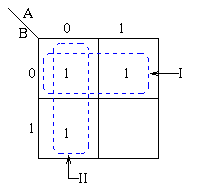Pairs of 1’s are grouped as shown above, and the simplified answer is obtained by using the following steps:

• Note that two groups can be formed for the example given above, bearing in mind that the largest rectangular clusters that can be made consist of two 1s. Notice that a 1 can belong to more than one group.
• The first group labelled I, consists of two 1s which correspond to A = 0, B = 0 and A = 1, B = 0. Put in another way, all squares in this example that correspond to the area of the map where B = 0 contains 1s, independent of the value of A. So when B = 0 the output is 1. The expression of the output will contain the term• For group labelled II corresponds to the area of the map where A = 0. The group can therefore be defined as. This implies that when A = 0 the output is 1. The output is therefore 1 whenever B = 0 and A = 0
• Hence the simplified answer is Z =+### 3-Variable Karnaugh Map

The truth table is shown first.  The Karnaugh Map for this truth table is shown after the truth table.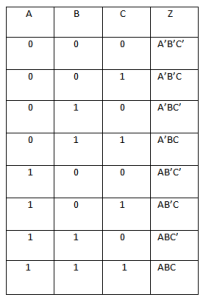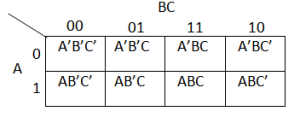The K-map for 3 variables is plotted above.

We can notice that the columns for 11 and 10 are inter-changed. This is done to allow only one variable to change across adjacent cells. This adjustment in columns allows in minimization of logic mapped into tables.

#### Example-1

Minimize following 3 variable function.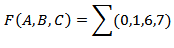Above is a common format of representing the K-map problems.

The numbers 0,1,6,7 are the location of cells in the 3-variable k-map table.

3 variable K– map with 1 and 0 values assigned to cells is shown in table below.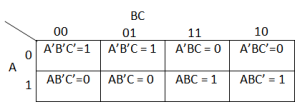Any adjacent 2, 4 or 8 cells can be grouped to find a minimized logic value.
Following plot will show grouping of adjacent cells.
The two step minimization equation is shown below.
With reference to the Karnaugh-map above the cells under the dotted box’s can be combined to come-up with following reduced  equation.

### 4-Variable Karnaugh Map

K-map for 4 variables x,y,z,w is discussed below with truth table and k-map plot.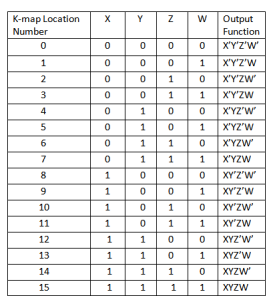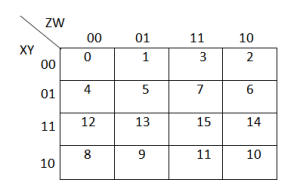#### Example:1

Minimize the following Boolean expression using K-map.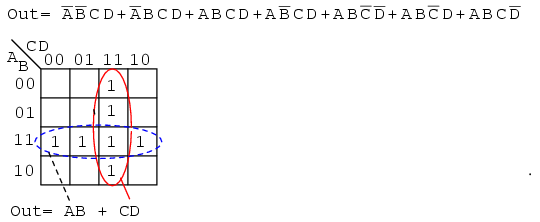The above Boolean expression has seven product terms. They are mapped top to bottom and left to right on the K-map above.

For example, the first term A’B’CD is first row 3rd cell, corresponding to map location A=0, B=0, C=1, D=1.

The other product terms are placed in a similar manner.

Encircling the largest groups possible, two groups of four are shown above.

The dashed horizontal group corresponds the the simplified product term AB.

The vertical group corresponds to Boolean CD.

Since there are two groups, there will be two product terms in the Sum-Of-Products result of Out=AB+CD.

#### Example:2

Minimize the following 4-variable function:#### Solution:

The numbers 0, 2, 4, 6, 12, 14 are the location of cells in the 4-var k-map table.

Shown below is a 4 variable K– map with 1 and 0 values assigned to cells.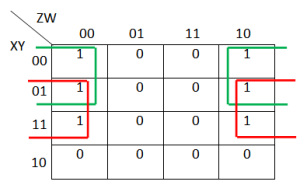The K-map for 4 variables is plotted above.

We can notice that the column and rows for 11 and 10 are inter-changed. This is done to allow only one variable to change across adjacent cells. This adjustment in columns allows in minimization of logic mapped into tables.

Any adjacent 1, 2, 4 or 8 cells can be grouped to find a minimized logic value.

With reference to the table above the cells under the green and red boxes can be combined to come up with following reduced  equation.

The group of four cells in green box corresponds to Boolean X’W’ and the group of  four cells in red box corresponds to Boolean YW’.

Hence the minimized function will be :